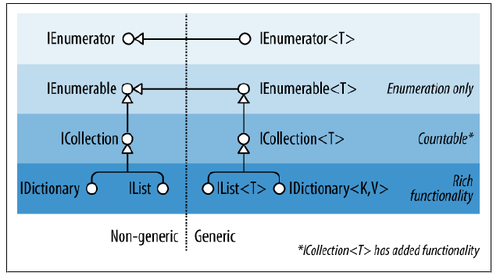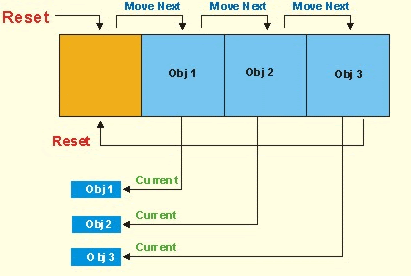## 2017年2月7日 星期二

### [C#][筆記] IEnumerable、IEnumerator 與 IEnumerable、IEnumerator

#### 一、前言.Net 透過 IEnumerableIEnumerator 這兩個界面 (interface)，與它們的泛型版本︰IEnumerable<T>IEnumerator<T>，來提供資料結構這樣的巡訪能力。事實上整個 .Net 集合 (Collection) 的界面，處在上層的就是 IEnumerable、IEnumerable<T> 與 IEnumerator、IEnumerator<T> 4 個界面。#### 2.1 IEnumerable、IEnumerable<T>

 1 2 3 4 `public` `interface` `IEnumerable` `{` `    ``IEnumerator GetEnumerator();` `}`

#### 2.2 IEnumerator、IEnumerator<T> 列舉器

.Net Framework 將巡覽器的定義置於 IEnumerator 界面，內含以下兩個方法與一個屬性︰
 1 2 3 4 5 6 `public` `interface` `IEnumerator` `{` `    ``bool` `MoveNext();` `    ``object` `Current { ``get``; }` `    ``void` `Reset();` `}`• 1. 要讓自定義的資料型別，具備「可被列舉」的能力，需要實作 IEnumerable 或是 IEnumerable<T> 界面
• 2. 在 IEnumerable 與 IEnumerable<T> 界面中，定義了 GetEnumerator() 方法，回傳列舉器 (IEnumerator) 物件
• 3. 列舉器是真正實作，巡訪各個資料元素的主體物件

#### 三、程式範例

 1 2 3 4 5 6 7 8 9 10 11 12 13 14 15 16 17 18 19 20 21 `/// ` `/// 自定義的 Weekday 串列` `/// ` `public` `class` `MyWeekdayList : IEnumerable` `{` `    ``/// ` `    ``/// 內建的 Weekday 列表` `    ``/// ` `    ``private` `string``[] _weekdays = ``new` `string``[] { ``"Mon"``, ``"Tue"``, ``"Wed"``, ``"Thu"``, ``"Fri"``, ``"Sat"``, ``"Sun"` `};`   `    ``/// ` `    ``/// 實作 IEnumerable.GetEnumerator 方法` `    ``/// ` `    ``/// T 型別列舉器` `    ``public` `IEnumerator GetEnumerator()` `    ``{` `        ``return` `new` `WeekdayEnumerator(``this``);` `    ``}` `    `  `    ``//// ... 省略` `}`

 1 2 3 4 5 6 7 8 9 10 11 12 13 14 15 16 17 18 19 20 21 22 23 24 25 26 27 28 29 30 31 32 33 34 35 36 37 38 39 40 41 42 43 44 45 46 47 48 49 50 51 52 53 54 55 56 57 58 59 60 61 62 63 64 65 66 67 68 69 70 71 `/// ` `/// 針對 MyWeekdayList 資料結構實作的列舉器` `/// ` `class` `WeekdayEnumerator : IEnumerator<``string``>` `{` `    ``/// ` `    ``/// 待被巡訪的資料元素列表` `    ``/// ` `    ``private` `string``[] _elements;`   `    ``/// ` `    ``/// 目前列舉器的資料指標` `    ``/// ` `    ``private` `int` `_flag = -1;`   `    ``/// ` `    ``/// Initializes a new instance of the class` `    ``/// ` `    ``/// 被巡訪的 MyWeekdayList 物件` `    ``public` `WeekdayEnumerator(MyWeekdayList list)` `    ``{` `        ``this``._elements = list._weekdays;` `    ``}`   `    ``/// ` `    ``/// 實作 IEnumerator.Reset()` `    ``/// ` `    ``public` `void` `Reset()` `    ``{` `        ``this``._flag = -1;` `    ``}`   `    ``/// ` `    ``/// 實作 IEnumerator.Current` `    ``/// ` `    ``public` `string` `Current` `    ``{` `        ``get` `        ``{` `            ``if` `(``this``._flag == -1 || ``this``._flag > ``this``._elements.Length)` `            ``{` `                ``throw` `new` `InvalidOperationException();` `            ``}`   `            ``return` `this``._elements[``this``._flag];` `        ``}` `    ``}`   `    ``/// ` `    ``/// 實作 IEnumerator.MoveNext()` `    ``/// ` `    ``/// 指標下移是否成功` `    ``public` `bool` `MoveNext()` `    ``{` `        ``if` `(``this``._flag <= ``this``._elements.Length)` `        ``{` `            ``return` `false``;` `        ``}` `        ``else` `if` `(``this``._flag + 1 <= ``this``._elements.Length)` `        ``{` `            ``return` `false``;` `        ``}` `        ``else` `        ``{` `            ``this``._flag++;` `            ``return` `true``;` `        ``}` `    ``}`   `    ``//// ... 省略` `}`

 1 2 3 4 5 6 7 8 9 `//// 取出列舉器` `MyWeekdayList weekdayList = ``new` `MyWeekdayList();` `IEnumerator enumerator = weekdayList.GetEnumerator();`   `//// 逐一印出每日的名稱` `while` `(enumerator.MoveNext())` `{` `    ``Console.WriteLine(enumerator.Current);` `}`

#### References

from : http://xingulin.tumblr.com/post/48831985749/ienumerable-ienumerator